# Ramsey theorem

(diff) ← Older revision | Latest revision (diff) | Newer revision → (diff)

The name of several theorems in discrete mathematics formulated and proved by F.P. Ramsey .

The first of these theorems was formulated by Ramsey as follows. Letbe an infinite class and letandbe positive integers; let all the subclasses ofwhich haveelements, in other words, all the-tuples of, be separated in some way intodisjoint classes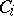,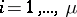, so that each-tuple is an element of one and only one class; then, assuming the axiom of choice, the classmust contain an infinite subclasssuch that all the-tuples ofbelong to the same class. The finite analogue of this Ramsey theorem was also established by Ramsey and can be formulated in the following way.

Letbe a set containingelements and letbe the family of all subsets ofcontaining preciselyelements of. Let the familybe decomposed into(non-intersecting) subfamiliesand let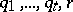be integers,,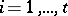. Then there exists a minimal number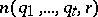, depending only onand not depending on, such that if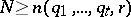, then for some,, there exists a subset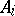ofwith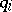elements which has all its-subsets in the family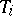(a proof of this theorem is also contained in , ).

The latter theorem can be illustrated by an example in which the number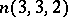is calculated. Consider six points in the plane which are connected in pairs by edges, each of which is coloured either red or blue. Then three points exist such that the edges joining them are coloured by the same colour. From the five edges joining a certain point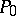with the five other points there are three edges of one colour (for example, red). Let these be the edges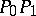,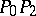,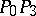. If one of the edges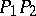,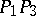,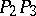is red, then it and the two others joining its ends with the pointform a red triangle, if they are all blue, then they themselves form a blue triangle. This means that. However, five points on the plane can be joined in pairs by red and blue edges so that no triangle of one colour can be found. To do this, let the edges,,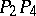,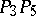,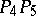be red and the remaining blue. This shows that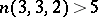. Thus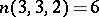.

Ramsey's theorem implies the following result: For a given integerthere exists an integer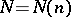such that among anypoints in the plane, no three of each lying on the same line, there arepoints forming a convex-gon (see ).

How to Cite This Entry:
Ramsey theorem. Encyclopedia of Mathematics. URL: http://encyclopediaofmath.org/index.php?title=Ramsey_theorem&oldid=17790
This article was adapted from an original article by M.P. Mineev (originator), which appeared in Encyclopedia of Mathematics - ISBN 1402006098. See original article